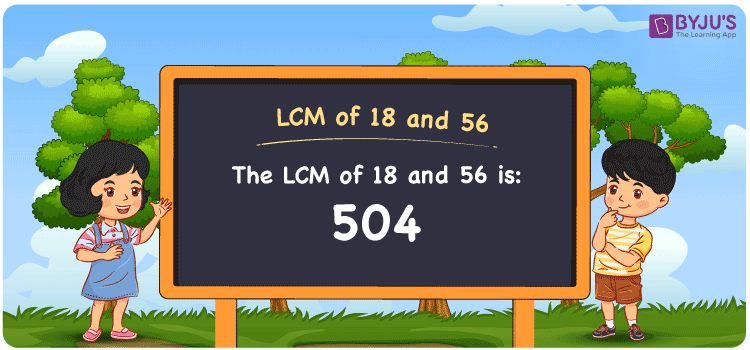# LCM of 18 and 56

LCM of 18 and 56 is 504. Students will learn how the least common multiple of 18 and 56 can be obtained among the common multiples. (18, 36, 54, 72, ….) and (56, 112, 168, 224, 280, 336, 392,….) are the multiples of 18 and 56. The methods used to find the LCM of two numbers are prime factorisation, by listing the multiples and division.

Also read: Least common multiple

## What is LCM of 18 and 56?

The answer to this question is 504. The LCM of 18 and 56 using various methods is shown in this article for your reference. The LCM of two non-zero integers, 18 and 56, is the smallest positive integer 504 which is divisible by both 18 and 56 with no remainder.## How to Find LCM of 18 and 56?

LCM of 18 and 56 can be found using three methods:

• Prime Factorisation
• Division method
• Listing the multiples

### LCM of 18 and 56 Using Prime Factorisation Method

The prime factorisation of 18 and 56, respectively, is given by:

18 = 2 x 3 x 3 = 2¹ x 3²

56 = 2 x 2 x 2 x 7 = 2³ x 7¹

LCM (18, 56) = 504

### LCM of 18 and 56 Using Division Method

We’ll divide the numbers (18, 56) by their prime factors to get the LCM of 18 and 56 using the division method (preferably common). The LCM of 18 and 56 is calculated by multiplying these divisors.

 2 18 56 2 9 28 2 9 14 3 9 7 3 3 7 7 1 7 x 1 1

No further division can be done.

Hence, LCM (18, 56) = 504

### LCM of 18 and 56 Using Listing the Multiples

To calculate the LCM of 18 and 56 by listing out the common multiples, list the multiples as shown below

Multiples of 18 = 18, 36, 54, 72, 90, 108, 126, 144, 162, 180, . . . ., 450, 468, 486, 504, . . . .

Multiples of 56 = 56, 112, 168, 224, 280, 336, 392, 448, 504, 560, . . . ., 280, 336, 392, 448, 504, . . . .

LCM (18, 56) = 504

## Video Lesson on Applications of LCM## LCM of 18 and 56 Solved Examples

Question: The LCM and GCD of two numbers are 504 and 2. If one number is 56, find the other.

Solution:

Given

LCM = 504

GCD = 2

Consider y as the other number

LCM x GCD = 56 x y

y = (LCM x GCD)/56

y = (504 x 2)/56

y = 18

Hence, the other number is 18.

## Frequently Asked Questions on LCM of 18 and 56

### In the numbers 5, 105, 504, 302, find the LCM of 18 and 56.

The smallest common multiple evenly divisible by 18 and 56 is the LCM value. The number 504 satisfies this condition.

### Find the GCF if the LCM of 18 and 56 is 504.

LCM x GCF = 18 x 56

Given

LCM of 18 and 56 = 504

504 x GCF = 1008

GCF = 1008/504 = 2

### Write the relation between GCF and LCM of 18 and 56.

The relation between GCF and LCM of 18 and 56 is

LCM x GCF = 18 x 56

LCM x GCF = 504

### What is the LCM of 18 and 56?

The LCM of 18 and 56 is 504.

### Mention the methods used to find the LCM of 18 and 56.

The methods used to find the LCM of 18 and 56 are prime factorisation, division and listing the multiples.